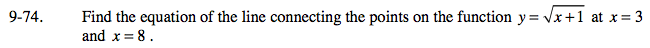### Home > CAAC > Chapter 16 > Lesson 16.9.2.1 > Problem9-74

9-74.Determine the y-coordinates for each of the points.

Find the slope between the two points.

Choose one of the points and use the slope.
Write the equation of the line in point-slope form.
(yy1) = m(xx1)